# Temporal Generative Adversarial Nets with Singular Value Clipping

Shunta Saito
(† Equal contributions)

Preferred Networks Inc.

ICCV 2017

[Code] [Paper] [Bibtex]

#### Abstract

In this paper, we propose a generative model, Temporal Generative Adversarial Nets (TGAN), which can learn a semantic representation of unlabeled videos, and is capable of generating videos. Unlike existing Generative Adversarial Nets (GAN)-based methods that generate videos with a single generator consisting of 3D deconvolutional layers, our model exploits two different types of generators: a temporal generator and an image generator. The temporal generator takes a single latent variable as input and outputs a set of latent variables, each of which corresponds to an image frame in a video. The image generator transforms a set of such latent variables into a video. To deal with instability in training of GAN with such advanced networks, we adopt a recently proposed model, Wasserstein GAN, and propose a novel method to train it stably in an end-to-end manner. The experimental results demonstrate the effectiveness of our methods.

#### Results on Video Generation

Following results are random samples generated by TGAN (not cherry picked).
 Moving MNIST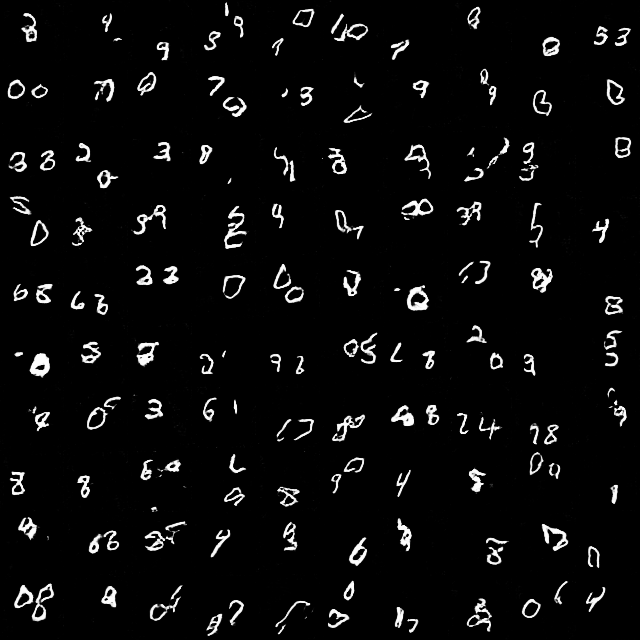UCF-101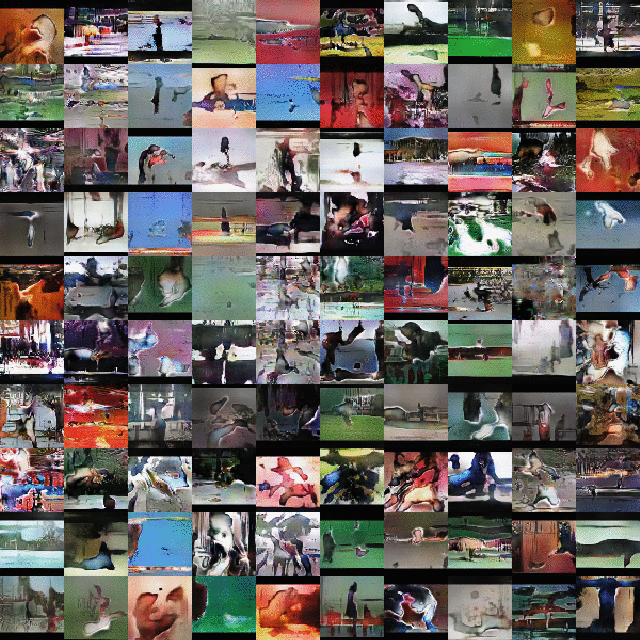UCF-101 (label conditional)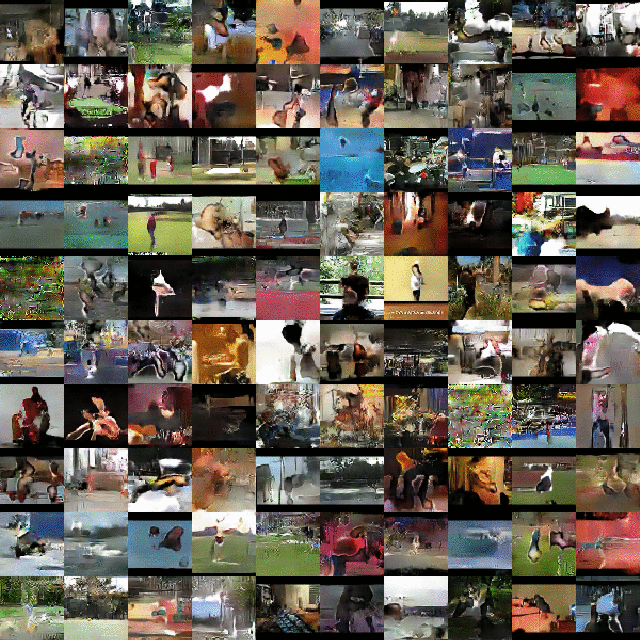Golf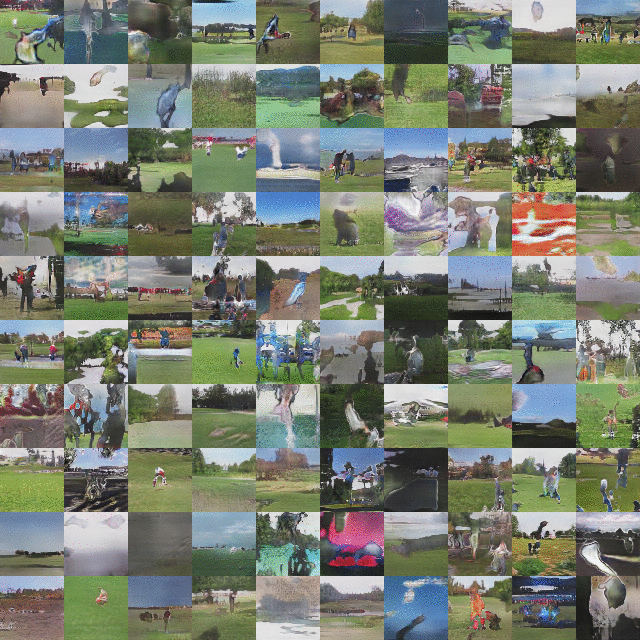#### Our Model

Our model consists of Generator and Discriminator alike usual GANs. Unlike previous video generating GANs using 3D convolution layers, we decompose the Generator into 1D convolution + 2D convolution as illustrates below: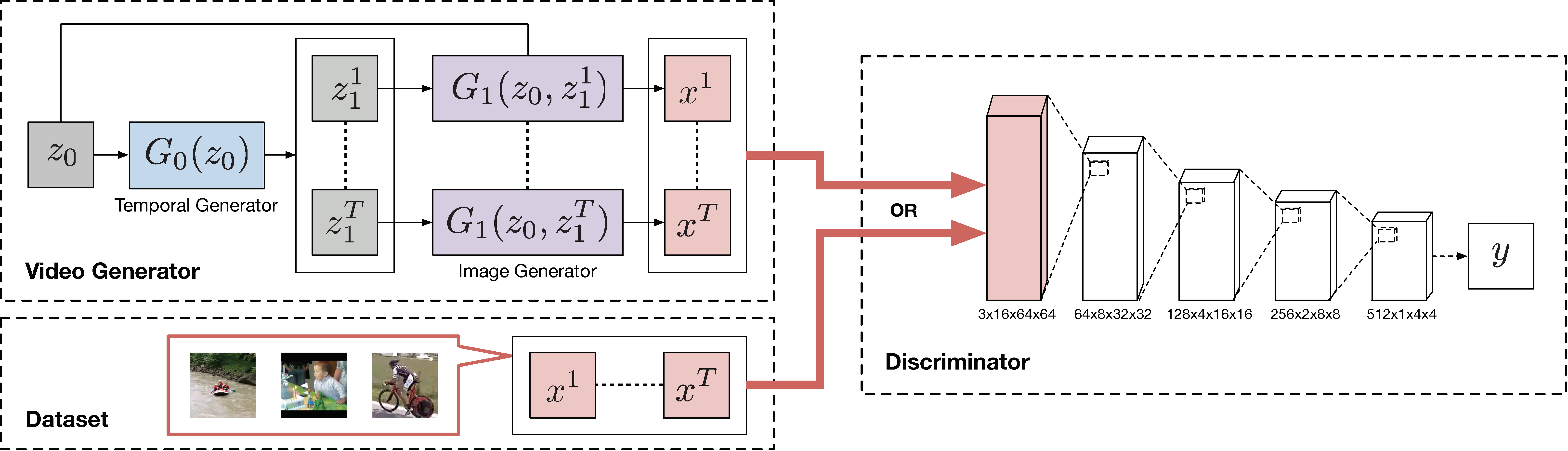The video generator consists of two generators, the temporal generator $$G_0$$ and the image generator $$G_1$$. The temporal generator $$G_0$$ yields a set of latent variables $$z^t_1(t = 1, . . . , T)$$ from $$z_0$$. The image generator $$G_1$$ transforms those latent variables $$z^t_1(t = 1, . . . , T)$$ and $$z_0$$ into a video data which has $$T$$ frames. The discriminator consists of three-dimensional convolutional layers, and evaluates whether these frames are from the dataset or the video generator.

#### Singular Value Clipping

We use Wasserstein GAN objective to train the model. WGAN requires the discriminator to fulfill the K-Lipschitz constraint, and the authors employed a parameter clipping method that clamps the weights in the discriminator to [−c, c]. However, we empirically observed that the tuning of hyper parameter c is severe, and it frequently fails in learning under a different situation like our proposed model.

Instead of the original WGAN, we add a constraint to all linear and convolutional layers in the discriminator that satisfies the spectral norm of weight parameter W is equal or less than one to satisfy K-Lipschitz constraint. This means that the singular values of weight matrix are all one or less. To implement this, we perform singular value decomposition after parameter update, replace all the singular values larger than one with one, and reconstruct the parameter with them, which we call Singular Value Clipping (SVC).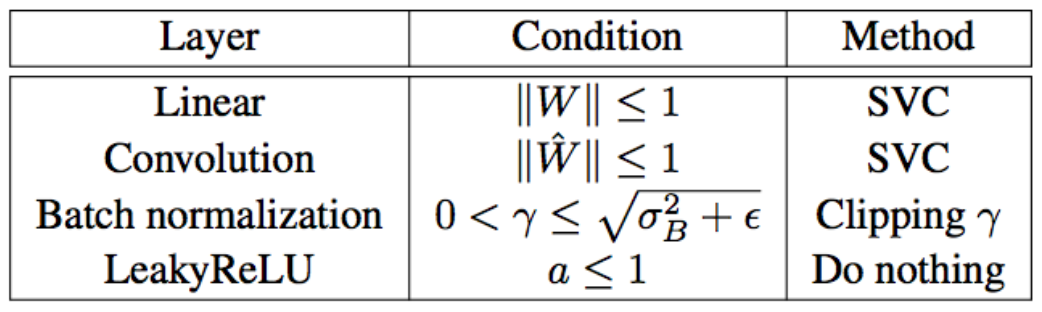#### Acknowledgements

We would like to thank Brian Vogel, Jethro Tan, Tommi Kerola, and Zornitsa Kostadinova for helpful discussions.